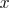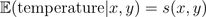Want to share your content on R-bloggers? click here if you have a blog, or here if you don't.As Mark Twain said “the
art of prophecy is very difficult, especially about the future
” (well, actually I am not sure Mark Twain was the  first one to say so,
but if you’re interested by that sentence, you can look here). I have been rather surprised to see how Canadians can
be interested in weather, and weather forecasts (see e.g. here for a
first study). For instance, on that website (here),
we can have forecasts of the temperature (but also rain and wind, which
is interesting when you ride a bike) over the next week, almost on an
hourly basis (I show here only GFS forecasts (Global Forecast System,here), which is quite standard, see here or there, but other meteorological models are considered on the website)As a statistician (or maybe more as a bike rider) I was wondering if I
should trust those forecasts… and if a mistake today means an error
tomorrow, too…
So, to look at this problem, Vincent (alias @Vicnent)
helped me (again, since he helped me already to get backups of betting
websites during the soccer world cup, in June) to make backups of those
tables…

• A descriptive study

For instance, if we look at forecasts made on the 21st of October (00:00) we have the following for the temperature in °C, for different time horizon,6 hours latter (21st of October (06:00)), we have the followingOn the other hand, consider forecasts made for the 25th of October (11:00) we havewhile for 3 hours later (25th of October (14:00)) we haveand again for 3 hours later (25th of October (17:00)) we haveSo obviously, 5 days earlier, there has been a bump of optimism, or
perhaps simply a typing mistake since we jump from 4 before and after
to 14 during 6 hours*.
If we can expect to have different predictions for different days (even
within the day since it is usually cooler during the night), we can try
to see if bumps, or forecast errors can be understood.
Here, it is difficult to observe the scatterplot of temperature,That graph was based on the following points, with here the scatterplot of prediction made on the 21st of October (00:00)with a smoothed version below (using standard splines),or, if we consider forecasts made for the 25th of October (11:00) we havewith again, a smoothed version,The code is the  following,

```donnees=read.table("http://perso.univ-rennes1.fr/
arthur.charpentier/pred-meteo2.txt")
x=donnees\$DATE1+donnees\$HEURE1/24
x[x<15]=x[x<15]+31
y=donnees\$DATE2+donnees\$HEURE2/24
y[y<15]=y[y<15]+31
z=y-x
h=donnees\$TEMPERATURE
D=data.frame(x,y,z,h)
X0=sort(unique(x))
Y0=sort(unique(y))
Z0=sort(unique(z))
matY.X=matrix(NA,length(X0),length(Y0))
library(splines)
for(i in 1:length(X0)){
dd=D[D\$x==X0[i],]
ax=dd\$y
ay=dd\$h
a=data.frame(ax,ay)
ra=lm(ay~bs(ax,df=5),data=ba)
X=Y0[(Y0>min(ax))&(Y0min(ax))&(Y0
So, let us look at levels of iso-temperature, as a function of the date the prediction is made (the x-axis) and the horizon (the y-axis). First, we we fix the date of the prediction, we get (from the code above)and then, when we fix the horizon of the predictionIt looks like the small heat wave
we experienced at the end of October has been perfectly predicted...
And here, it looks like the only pattern we can observe is the standard evolution of temperature: there are no bumps due to forecasting problems..

Modeling predicted temperatures

Let us use some additive or multiplicative models, i.e. eitherwheredenotes the date the prediction was made andthe date the horizon, orThis
was easy with the gam framework... For instance, consider the following
code (some regressions mentioned below will be used later on)
library(mgcv)
library(splines)
REG1=gam(h~s(x,y),data=D,family=gaussian)
REG2=lm(h~bs(x)+bs(y),data=D)
REG3=gam(h~s(x)+s(y),data=D,family=gaussian)
REG5=gam(h~s(y),data=D,family=gaussian)
D\$e=residuals(REG5)
REG6=gam(e~s(x,y),data=D,family=gaussian)and for the multiplicative model(colors are different because of the log scaling but the shape is similar).
For the component on(in blue), we have, with the additive modeland for the multiplicative modelOn the other hand, for the component on(in red) we have, with the additive modeland for the multiplicative modelNote that we observe something which looks like the true temperature (we actually had)So
finally, on average, those predictions are consistent with the true
temperature we experienced. But so far, we cannot say anything about prediction
errors. If we consider a bivariate spline regression, i.e.for some smoothed function, we have, again, the same kind of predictions,It
looks like what we've seen before. And because we have those horizontal
lines, only the horizonshould be considered.... So let us study the
following modelwhere we obtain the following prediction(which is similar with previous graph) but if we fit a spline on residuals obtained from that regression, i.e.wherewe have the following distribution forThis
is where we can see prediction errors. We can observe that meteorologists
have been a bit surprised by the heat-wave we observed just after the
25th. But let us wait a few more days to get more data, and perhaps
we'll have a look at something that I do care about: prediction of rainfall....
*
perhaps I should mention that we do not have backups of tables, but backups of
html pages... then I use simple linguistic tools available on R to
extract the information where it should be....

var vglnk = {key: '949efb41171ac6ec1bf7f206d57e90b8'};
(function(d, t) {
var s = d.createElement(t);
s.type = 'text/javascript';
s.async = true;
// s.defer = true;
var r = d.getElementsByTagName(t);
r.parentNode.insertBefore(s, r);
}(document, 'script'));

Related
ShareTweet